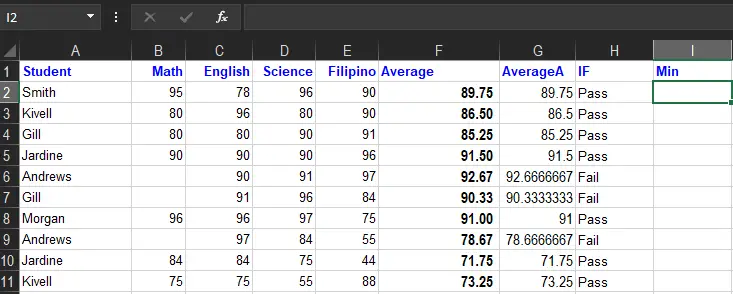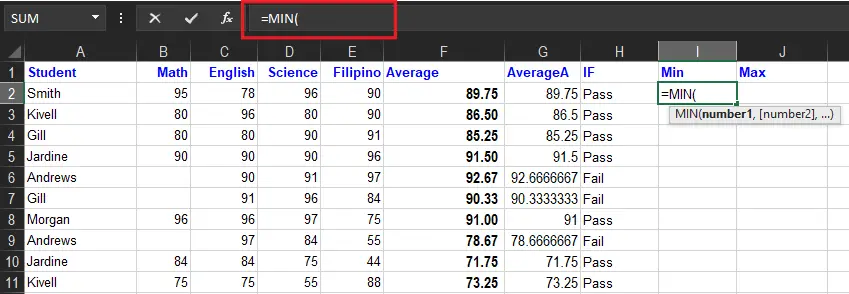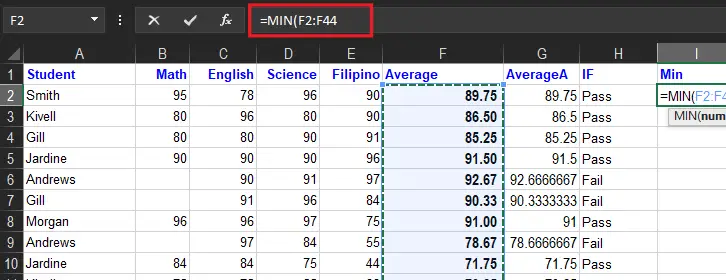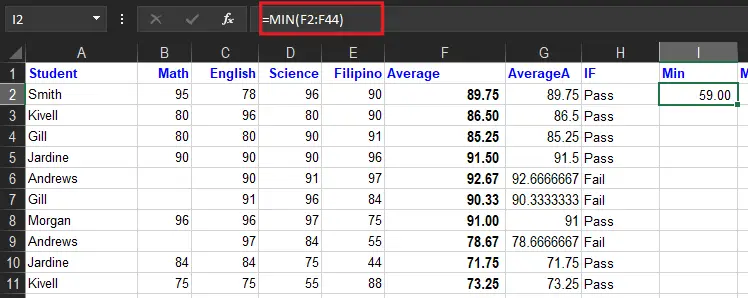# Min Function in Excel Example Ways To Use

Today in this tutorial we will describe how to use MIN function of Microsoft Excel in finding the lowest value of a condition. As we are going to highlight and explain for better understanding.

Moreover this tutorial, we’ll learn the basic but quite important part of MIN function. As this article will provide ways to yields the number excluding zero values, smallest value and its absolute minimum value based on criteria.

Now, let get started!

## what is min function in excel

The same with other functions this MIN function is also belong to the Excel Statistical functions. It ask find the minimum value present on the given list of arguments , such as a set of numeric values that returns the smallest value.

In addition to this the MIN function ignores the numbers text and logical values True and False as well as text values unlike MINA function does otherwise.

In financial aspect MIN function is valuable in analyzing and calculating debt and deminishment schedules. For instance, MIN is able to return the smallest number, fastest time in a race, the earliest date, the smallest percentage, the lowest temperature, or the bottom sales number.

Furthermore, the arguments a Min function can handle takes up to 225 of total in the form number1, number2, number3, etc. The good thing is it could be hardcoded constant, cell reference, or orange or the combination of it.

## Syntax

`=MIN(number1, [number2], ...)`

## Arguments

• `number1 `– Number, reference to numeric value, or range that contains numeric values.
• `number2 `– [optional] Number, reference to numeric value, or range that contains numeric values.

## Return Value

The min function returns the smallest value supplied data

## how to use min function in excel

Time needed: 3 minutes

Here is the steps How to use the `=MIN` formula:

1. Select a cell I22. Type =MIN3. Double click the MIN command

4. Select a range (F2:F44)5. Hit enter to get the expected result.As you noticed the value that MIN function retuns is smallest value present in the data. To make it clear take a look this example formula:

```=MIN(70, 80, 75, 89, 95)
So the value it will return is 70.```

Here is another example which accept values as separate arguments or in ranges or arrays:

```=MIN(5,10)
=MIN(A1,A2,A3)
=MIN(A1:A10)
=MIN(A1:A10,C1:C10)```

Take a look this formula that returns with minimum criteria.

MIN ignores logical values and numbers entered as text, unless they are provided as arguments

```=MIN(5,TRUE)

It means it will return 1 based on the criteria.
=MIN(7,5,"3")

It means it will return 3 based on the criteria.
```

Tips: In retrieving the nth nth smallest value in a data set, use the SMALL function. Besides use RANK function to determine a number’s rank in a data set.

## min function in excel with condition

This time let’s examine the MIN function with a condition. Supposedly we will determine the smallest grade that a students have with the criteria given.

Apparently, when making a conditions in Excel it is accompanied by the IF function of Excel. All you need to do is make a perfect combination of MIN and IF to solve this task:

There you go let’s use the formula below.

`{=MIN(IF(A2:A44=`“jones”`,F2:F44))} `

Determine minimum by criteria using MIN IF Excel formulas

Note: Press Ctrl + Shift + Enter so that array function to work and enjoy.

## max min function excel

There are some essential functions that every user should know, and the MAX and MIN functions are two of them. The MAX function allows you to find the highest number in a given range, while the MIN function allows you to find the lowest number in a given range.

This quick tutorial will cover the basics of how to apply both functions.

## Summary

In summary we learned the Min Function Excel Example Ways To Use. So here are to keep in mind after the discussion.

• Arguments can be numbers, names, arrays, or references.
• Excel MIN ignores empty cells, text values, and TRUE and FALSE in references.
• Excel MIN will consider text representations of numbers and logical values supplied directly as arguments.
• To include logical values in a reference, see the MINA function.
• MIN accepts up to 255 arguments. If arguments contain no numbers, MIN returns 0.